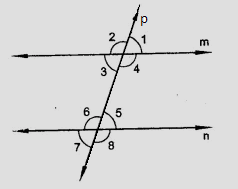"
">

# In the figure, transversal $l$, intersects two lines $m$ and $n, \angle 4 = 110^o$ and $\angle 7 = 65^o$. Is $m \parallel n$?"

Given:

Transversal $l$, intersects two lines $m$ and $n, \angle 4 = 110^o$ and $\angle 7 = 65^o$.

To do:

We have to find whether $m \parallel n$.

Solution:

$\angle 4 = 110^o$ and $\angle 7 = 65^o$

$\angle 5 = \angle 7 = 65^o$                       (Vertically opposite angles)

$\angle 4 + \angle 5 = 110^o + 65^o$

$= 175^o$

Here, $\angle 4$ and $\angle 5$ are co-interior angles.

The sum of co-interior angles $\angle 4$ and $\angle 5$ is not $180^o$.

Therefore,

$m$ is not parallel to $n$.

Updated on: 10-Oct-2022

31 Views